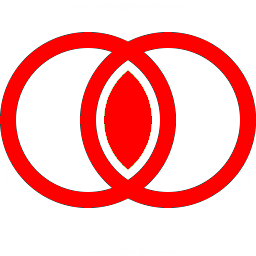INTERSECT_TWO_SURFACESUse the INTERSECT_TWO_SURFACES operation to compute the curve of intersection between two surfaces. This operation uses an iterative numerical method to approximate the curve of intersection. Four user-defined seed values (between 0 and 1) are required for the first iteration. Click the icon and then click on any control point of the first surface in one of the orthogonal projection views. Note that the control point will be highlighted in green. Then click on the second surface. (Note that self-intersections are not implemented; only the intersection of two different surfaces can be calculated). A dialog will be displayed with spinners for the four user-defined seed values. When you have selected the desired values, click OK. Calculation of the iterative algorithm is performed in a worker thread. When the calculation is complete, the curve of intersection is displayed in red in all four views. Note that this operation is only implemented for Bezier surfaces and Catmull-Rom surfaces. If one or more of the surfaces the user selects is a Linear surface, then the calculation is terminated. The INTERSECT_TWO_SURFACES mode is automatically disabled after the computation is complete. INTERSECT_TWO_SURFACES mode is not undoable.
 For the dedicated staff at Hammersmith Hospital. home